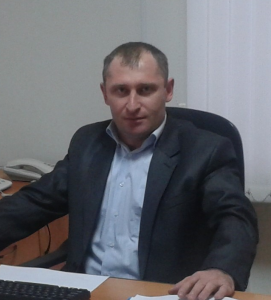# Bulletin KRASEC. Phys. & Math. Sci, 2015, V. 11, №. 2, pp. 24-35. ISSN 2313-0156

Back to contents

DOI: 10.18454/2313-0156-2015-11-2-24-35

MSC 35M10

ON MODEL OF LOADED HYPERBOLIC-PARABOLIC PARTIAL DIFFERENTIAL EQUATION OF SECOND ORDER

Khubiev K.U.

Institute of Applied Mathematics and Automation, 360000, Republic of Kabardino-Balkariya, Nalchik, st. Shortanova, 89a.

E-mail: olesya.masaeva@yandex.ru.

The paper investigates models of loaded mixed hyperbolic-parabolic equations with characteristic and noncharacteristic change of the type. For the proposed equation models, boundary value problems are considered, and explicit solutions are worked out.

Key words: equation model, loaded equation, hyperbolic-parabolic equation, boundary value problem.

References

1. Nakhushev A.M. Ob odnom priblizhennom metode resheniya kraevykh zadach dlya differentsial’nykh uravnenii i ego prilozheniya k dinamike pochvennoi vlagi i gruntovykh vod [On one aproximation method of solution boundary value problems for differential equations and its application to the dynamics of soil moisture and ground water]. Differentsial’nye Uravneniya – Differential Equations, 1982, V. 18,
No 1. pp. 72–81.
2. Nakhushev A.M. O zadache Darbu dlya odnogo vyrozhdayushchegosya nagruzhennogo integro-differentsial’nogo uravneniya vtorogo poryadka [Darboux problem for one degenerated loaded integral-differential equation of second order].Differentsial’nye Uravneniya – Differential Equations, 1976, V. 12, № 1. pp. 103–108.
3. Ozturk I. Boundary value problem for the loaded differential equation of fractional order. Doklady Adygskoi (Cherkesskoi) Mezhdunarodnoi Akademii Nauk, 1995, V. 1, № 2. pp. 12–17.
4. Tokova A.A. O pervoi kraevoi zadache dlya odnogo nagruzhennogo differentsial’nogo uravneniya vtorogo poryadka [On the first boundary value problem for one loaded differential equation of second order]. Doklady Adygskoi (Cherkesskoi) Mezhdunarodnoi Akademii Nauk, 2005. V. 8, № 1, pp. 87–91.
5. Tokova A.A. Ob odnoi nelokal’noi kraevoi zadache dlya nagruzhennogo differentsial’nogo uravneniya so znakoperemennoi kharakteristicheskoi formoi [On one nonlocal boundary value problem for a loaded differential equation with alternating characteristic form]. Vestnik Samarskogo gosudarstvennogo tekhnicheskogo universiteta. Seriya: Fiziko-matematicheskie nauki, 2011, № 2, pp. 40–45.
6. Nakhushev A.M. Nagruzhennye uravneniya i ikh primeneniya [Loaded equations and their applications]. Moscow, Nauka, 2012, 232 p.
7. Khubiev K.U. Ob odnoi modeli nagruzhennogo giperbolo-parabolicheskogo uravneniya v chastnykh proizvodnykh vtorogo poryadka s kharakteristicheskim izmeneniem tipa [On one model of a loaded
hiperbolic-parabolic partial differential euqation of second order with characteristic change of the type]. Doklady Adygskoi (Cherkesskoi) Mezhdunarodnoi Akademii Nauk, 2015, V. 17, № 2, pp. 48–51.
8. Tikhonov A.N., Samarskii A.A. ravneniya matematicheskoi fiziki [Mathematical physics equations]. Moscow, Nauka, 1977, 735 p.
9. Nakhushev A.M. Drobnoe ischislenie i ego primenenie [Fractional calculus and its application]. Moscow, Fizmatlit, 2003. 272 p.

For citation: Khubiev K.U. On model of loaded hyperbolic-parabolic partial differential equation of second order. Bulletin KRASEC. Physical and Mathematical Sciences 2015, vol. 11, issue 2, 24-35. DOI: 10.18454/2313-0156-2015-11-2-24-35.

Original article submitted: 12.09.2015Khubiev Kazbek Uzeirovich – Ph.D. (Phys. & Math.), Senior Researcher of Dep. Equations of mixed type, Institute of Applied Mathematics and Automation, Kabardino-Balkaria, Nalchik.